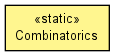org.shared.stat.util

## Class Combinatorics• ```public class Combinatorics
extends Object```
A static utility class for combinatorics.
• ### Field Summary

Fields
Modifier and Type Field and Description
`protected static double[]` `gammaLnCoefficients`
A lookup table of coefficients in support of `gammaLn(double)`.
• ### Method Summary

Methods
Modifier and Type Method and Description
`static double` `gamma(double x)`
`static double` `gammaLn(double x)`
The log-gamma function.
`protected static void` ```orderedPartition(DynamicObjectArray<int[]> acc, int[] sizes, int nSizes, int nRemaining)```
A helper method in support of `orderedPartition(int, int, int)`.
`static int[][]` `orderedPartition(int n)`
`static int[][]` ```orderedPartition(int n, int nPartsLower, int nPartsUpper)```
Calculates all ordered partitions of an `n` element set into at least `nPartsLower` parts and at most (exclusive) `nPartsUpper` parts.
`protected static void` ```partition(DynamicObjectArray<int[]> acc, int[] sizes, int nSizes, int currentSize, int nRemaining)```
A helper method in support of `partition(int, int, int)`.
`static int[][]` `partition(int n)`
`static int[][]` ```partition(int n, int nPartsLower, int nPartsUpper)```
Calculates all partitions of an `n` element set into at least `nPartsLower` parts and at most (exclusive) `nPartsUpper` parts.
• ### Methods inherited from class java.lang.Object

`clone, equals, finalize, getClass, hashCode, notify, notifyAll, toString, wait, wait, wait`
• ### Field Detail

• #### gammaLnCoefficients

`protected static final double[] gammaLnCoefficients`
A lookup table of coefficients in support of `gammaLn(double)`.
• ### Method Detail

• #### partition

`public static final int[][] partition(int n)`
Parameters:
`n` - the number of elements.
Returns:
the partitions.
• #### partition

```public static final int[][] partition(int n,
int nPartsLower,
int nPartsUpper)```
Calculates all partitions of an `n` element set into at least `nPartsLower` parts and at most (exclusive) `nPartsUpper` parts.
Parameters:
`n` - the number of elements.
`nPartsLower` - the lower bound on the number of parts.
`nPartsUpper` - the upper bound (exclusive) on the number of parts.
Returns:
the partitions.
• #### partition

```protected static final void partition(DynamicObjectArray<int[]> acc,
int[] sizes,
int nSizes,
int currentSize,
int nRemaining)```
A helper method in support of `partition(int, int, int)`.
Parameters:
`acc` - the partition accumulator.
`sizes` - the partition sizes.
`nSizes` - the number of parts so far.
`currentSize` - the part size so far.
`nRemaining` - the number of remaining elements.
• #### orderedPartition

`public static final int[][] orderedPartition(int n)`
Parameters:
`n` - the number of elements.
Returns:
the ordered partitions.
• #### orderedPartition

```public static final int[][] orderedPartition(int n,
int nPartsLower,
int nPartsUpper)```
Calculates all ordered partitions of an `n` element set into at least `nPartsLower` parts and at most (exclusive) `nPartsUpper` parts.
Parameters:
`n` - the number of elements.
`nPartsLower` - the lower bound on the number of parts.
`nPartsUpper` - the upper bound (exclusive) on the number of parts.
Returns:
the ordered partitions.
• #### orderedPartition

```protected static final void orderedPartition(DynamicObjectArray<int[]> acc,
int[] sizes,
int nSizes,
int nRemaining)```
A helper method in support of `orderedPartition(int, int, int)`.
Parameters:
`acc` - the ordered partition accumulator.
`sizes` - the ordered partition sizes.
`nSizes` - the number of parts so far.
`nRemaining` - the number of remaining elements.
• #### gamma

`public static final double gamma(double x)`
Parameters:
`x` - the input value.
Returns:
the gamma function evaluation.
• #### gammaLn

`public static final double gammaLn(double x)`
The log-gamma function.
Parameters:
`x` - the input value.
Returns:
the log-gamma function evaluation.